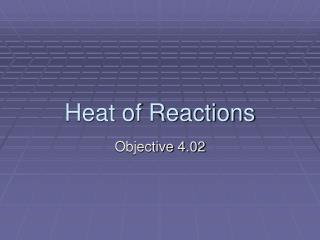DownloadDownload PresentationHeat of Reactions

# Heat of Reactions

Download Presentation## Heat of Reactions

- - - - - - - - - - - - - - - - - - - - - - - - - - - E N D - - - - - - - - - - - - - - - - - - - - - - - - - - -
##### Presentation Transcript

1. Heat of Reactions Objective 4.02

2. Specific Heat • Amount of energy required to raise the temperature of 1g of a substance by 1 degree Celsius (or Kelvin). • Cp = J/(g∙K) or J/(g∙°C) q = mCpΔT q = energy lost or gained m = mass ΔT = change in temp Ex: pg 513

3. Heat of Vaporization • The amount of heat required to vaporize 1g of liquid (water) at its boiling point. • Hv = (J/g) q = mHv

4. Heat of Fusion • The amount of energy required to melt one g of a solid (water) at its melting point. • Hf = (J/g) q = mHf